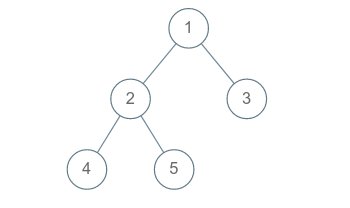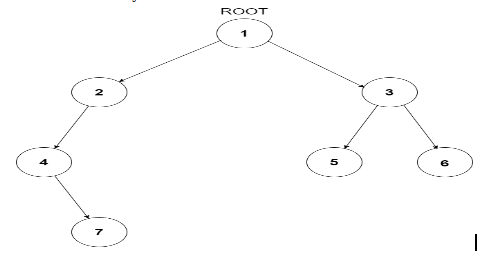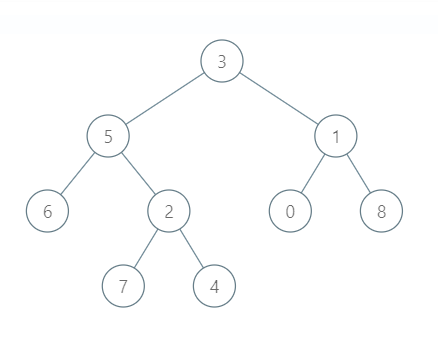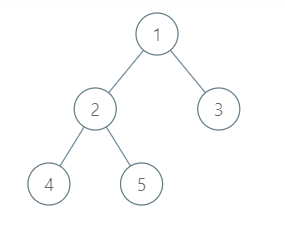Problem title
Difficulty
Avg time to solve

Maximum profit
Moderate
40 mins
Root to Leaf Path
Moderate
25 mins
Vertical Order Traversal
Moderate
35 mins
Print Permutations
Moderate
--
Merge Point of Two Linked Lists
Moderate
--
Justice league
Moderate
30 mins
Ninja And Trains
Hard
60 mins
Game of 3
Moderate
--
Ninja And Numbers
Moderate
40 mins
Similar Strings
Ninja
70 mins

# Root to Leaf Path

Difficulty: MEDIUM
Avg. time to solve
25 min
Success Rate
70%

Problem Statement

#### Note :

``````A leaf of a Binary Tree is the node which does not have a left child and a right child.
``````
##### For Example :
``````Given a binary tree :
````````````All the root to leaf paths are :
1 2 4
1 2 5
1 3
``````

#### Note :

``````1. Two nodes may have the same value associated with it.
2. The root node will be fixed and will be provided in the function.
3. Note that the nodes in a path will appear in a fixed order. For example, 1 2 3 is not the same as 2 1 3.
4. Each path should be returned as a string consisting of nodes in order and separated by a space.
5. The path length may be as small as ‘1’.
``````
##### Input Format :
``````The first line of the input contains a single integer 'T', representing the number of test cases.

The first line of each test case contains an integer 'N', which denotes the number of nodes in the tree.

The second line of each test case will contain the values of the nodes of the tree in the level order form ( -1 for 'NULL' node) Refer to the example for further clarification.
``````
##### Example :
``````Consider the binary tree
````````````The input of the tree depicted in the image above will be like :

1
2 3
4 -1 5 6
-1 7 -1 -1 -1 -1
-1 -1

Explanation :
Level 1 :
The root node of the tree is 1

Level 2 :
Left child of 1 = 2
Right child of 1 = 3

Level 3 :
Left child of 2 = 4
Right child of 2 = null (-1)
Left child of 3 = 5
Right child of 3 = 6

Level 4 :
Left child of 4 = null (-1)
Right child of 4 = 7
Left child of 5 = null (-1)
Right child of 5 = null (-1)
Left child of 6 = null (-1)
Right child of 6 = null (-1)

Level 5 :
Left child of 7 = null (-1)
Right child of 7 = null (-1)

The first not-null node (of the previous level) is treated as the parent of the first two nodes of the current level. The second not-null node (of the previous level) is treated as the parent node for the next two nodes of the current level and so on.

The input ends when all nodes at the last level are null (-1).
``````
##### Output Format :
``````For each test case, print all the root to leaf path nodes.
``````
##### Note :
``````You do not need to print anything, it has already been taken care of. Just implement the given function.
``````
##### Constraints :
``````1 <= T <= 10^2
1 <= N <= 3*10^3

Time Limit : 1 sec
``````
##### Sample Input 1 :
``````2
9
3 5 1 6 2 0 8 -1 -1 7 4 -1 -1 -1 -1 -1 -1 -1 -1
5
1 2 3 4 5 -1 -1 -1 -1 -1 -1
``````
##### Sample Output 1 :
``````3 5 6
3 1 0
3 1 8
3 5 2 7
3 5 2 4
1 3
1 2 4
1 2 5
``````

#### Explanation for Sample Input 1:

``````Test Case 1:
````````````Here root is 3 and 6, 7, 4, 0, 8 are leaf nodes
Path from 3 to 6 is 3 5 6
Path from 3 to 7 is 3 5 2 7
Path from 3 to 4 is 3 5 2 4
Path from 3 to 0 is 3 1 0
Path from 3 to 8 is 3 1 8

Test Case 2:
````````````Here root is 1 and 4, 5 are leaf nodes
Path from 1 to 4 is 1 2 4
Path from 1 to 5 is 1 2 5
``````
##### Sample Input 2 :
``````1
7
1 2 3 4 -1 5 6 -1 7 -1 -1 -1 -1 -1 -1
``````
##### Sample Output 2 :
``````1 3 5
1 3 6
1 2 4 7
``````Console